# How to Use Descartes' Rule of Signs (With Examples)

• Author:
• Updated date:

Ray is a licensed engineer in the Philippines. He loves to write about mathematics and civil engineering.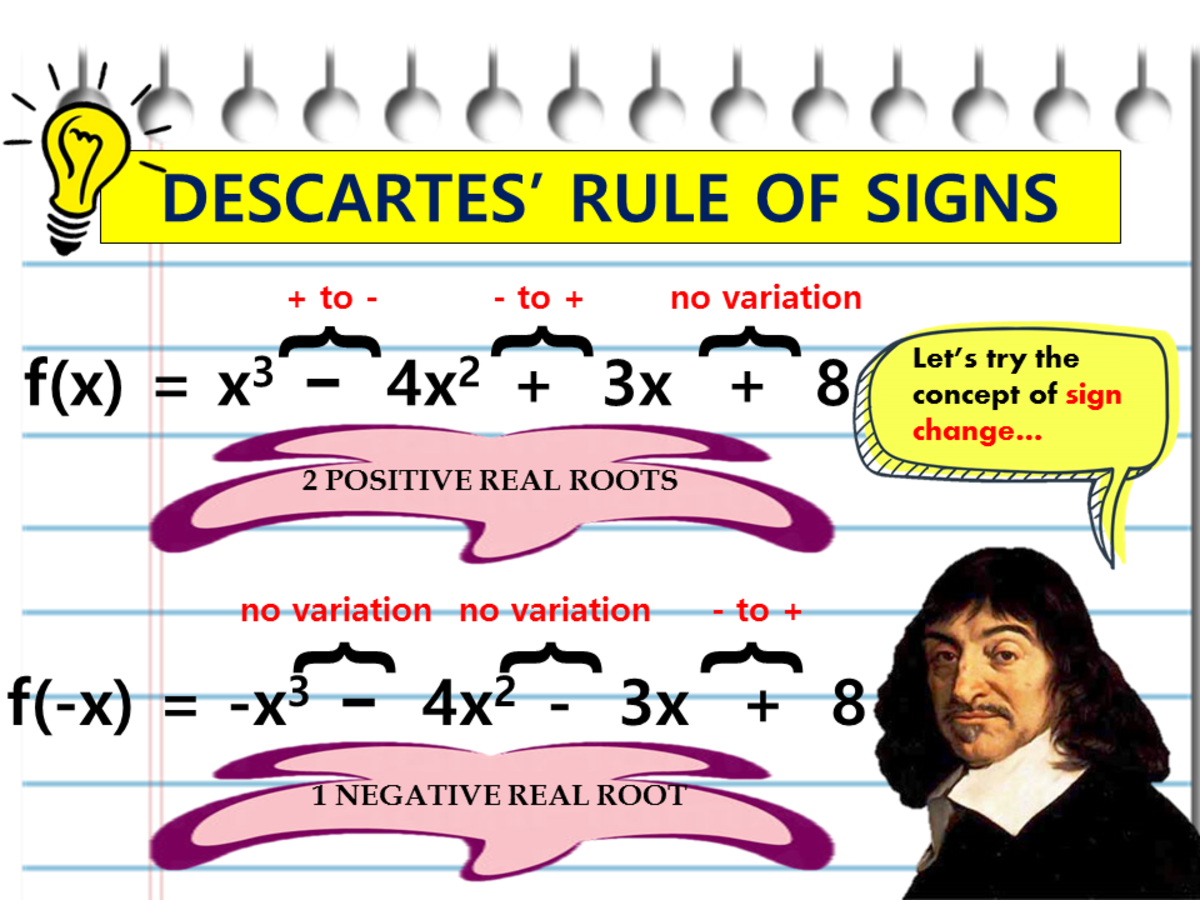Descartes' Rule of SignsJohn Ray Cuevas

## What Is Descartes' Rule of Signs?

Descartes’ Rule of Signs is a useful and straightforward rule to determine the number of positive and negative zeros of a polynomial with real coefficients. It was discovered by the famous French mathematician Rene Descartes during the 17th century. Before stating Descartes’ rule, we must explain what is meant by a variation of sign for such a polynomial.

If the arrangement of the terms of a polynomial function f(x) are in order of descending powers of x, we say that a variation of sign occurs whenever two successive terms have opposite signs. When counting the total number of variations of the sign, ignore the missing terms with zero coefficients. We also assume that the constant term (the term that does not contain x) is different from 0. We say there is a variation of sign in f(x) if two consecutive coefficients have opposite signs, as stated earlier.

## Step-by-Step Procedure on How to Use Descartes' Rule of Signs

Shown below are the steps in using Descartes' Rule of Signs.

1. Have an exact look at the sign of each term in the polynomial. Being able to identify the signs of the coefficients allows keeping track of the change in sign easily.
2. In determining the number of real roots, make the polynomial equation in the form P(x) for positive real roots and P(-x) for the negative real roots.
3. Look for the significant sign changes, which can go from positive to negative, negative to positive or no variation at all. A change in a sign is the condition if the two signs of adjacent coefficients alternate.
4. Count the number of sign variations. If n is the number of variations in sign, then the number of positive and negative real roots may equal to n, n-2, n-4, n-6, and so on. Remember to keep subtracting it by some multiple of 2. Stop subtracting until the difference becomes 0 or 1.

For instance, if P(x) has n = 8 number of sign variation, the possible number of positive real roots will be 8, 6, 4, or 2. On the other hand, if P(-x) has n = 5 number of changes in the sign of the coefficients, the possible number of negative real roots is 5, 3, or 1.

## Descartes' Rule of Signs Definition

Let f(x) be a polynomial with real coefficients and a non-zero constant term.

• The number of positive real zeros of f(x) either is equal to the number of variations of sign in f(x) or is less than that number by an even integer.

The number of negative real zeros of f(x) either is equal to the number of variations of sign in f(−x) or is less than that number by an even integer. Descartes’ Rule of Signs stipulates that the constant term of the polynomial f(x) is different from 0. If the constant term is 0, as in the equation x4−3x2+2x2−5x=0, we factor out the lowest power of x, obtaining x (x3−3x2+2x−5) = 0. Thus, one solution is x=0, and we apply Descartes’ rule to the polynomial x3−3x2+2x−5 to determine the nature of the remaining three solutions.

When applying Descartes’ rule, we count roots of multiplicity k as k roots. For example, given x2−2x+1=0, the polynomial x2−2x+1 has two variations of the sign, and hence the equation has either two positive real roots or none. The factored form of the equation is (x−1)2=0, and thus 1 is a root of multiplicity 2.

To illustrate the variety of signs of a polynomial f(x), here are some examples on the Descartes’ Rule of Signs.

## Example 1

### Finding the Number of Sign Variations in a Positive Polynomial Function

Using the Descartes’ Rule, how many variations in the sign are there in the polynomial f(x) = 2x5−7x4+3x2+6x−5?

### Solution

The signs of the terms of this polynomial arranged in descending order are shown below. Next, count and identify the number of changes in the sign for the coefficients of f(x). Here are the coefficients of our variable in f(x).

+2 -7 +3 + 6 -5

Scroll to Continue

## Read More From Owlcation

We have the first change in signs between the first two coefficients, the second change between the second and third coefficients, no change in signs between the third and fourth coefficients, and the last change in signs in between the fourth and fifth coefficients. Therefore, we have got one variation from 2x5 to −7x4, a second from −7x4 to 3x2, and a third from 6x to −5.

The given polynomial f(x) has three sign variations, as indicated by the braces.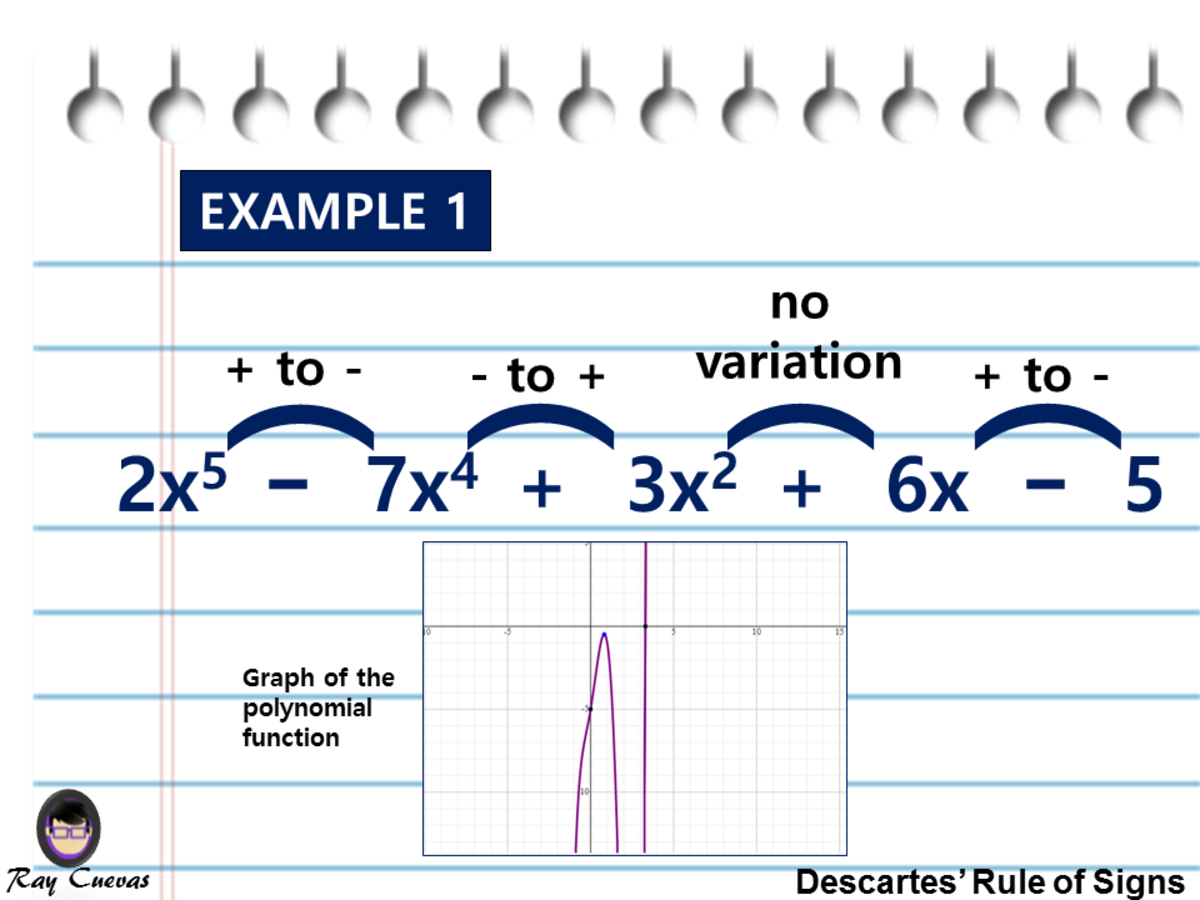Example 1: Finding the Number of Sign Variations in a Positive Polynomial Function Using Descartes' Rule of SignsJohn Ray Cuevas

## Example 2: Finding the Number of Sign Variations in a Negative Polynomial Function

Using the Descartes’ Rule, how many variations in the sign are there in the polynomial f(−x) = 2x5−7x4+3x2+6x−5?

Solution

Descartes' Rule in this example refers to the variations of sign in f(-x). Using the previous illustration in Example 1, simply the given expression using –x.

f(-x) = 2(-x)5 - 7(-x)4 + 3(-x)2 + 6(-x) – 5

f(-x) = -2x5 – 7x4 + 3x2 – 6x – 5

The signs of the terms of this polynomial arranged in descending order are shown below. Next, count and identify the number of changes in sign for the coefficients of f(-x). Here are the coefficients of our variable in f(-x).

-2 -7 +3 - 6 -5

The figure shows the variation from -7x4 to 3x2 and a second term 3x2 to -6x.

Hence, as indicated in the illustration below, there are two variations of sign in f(-x).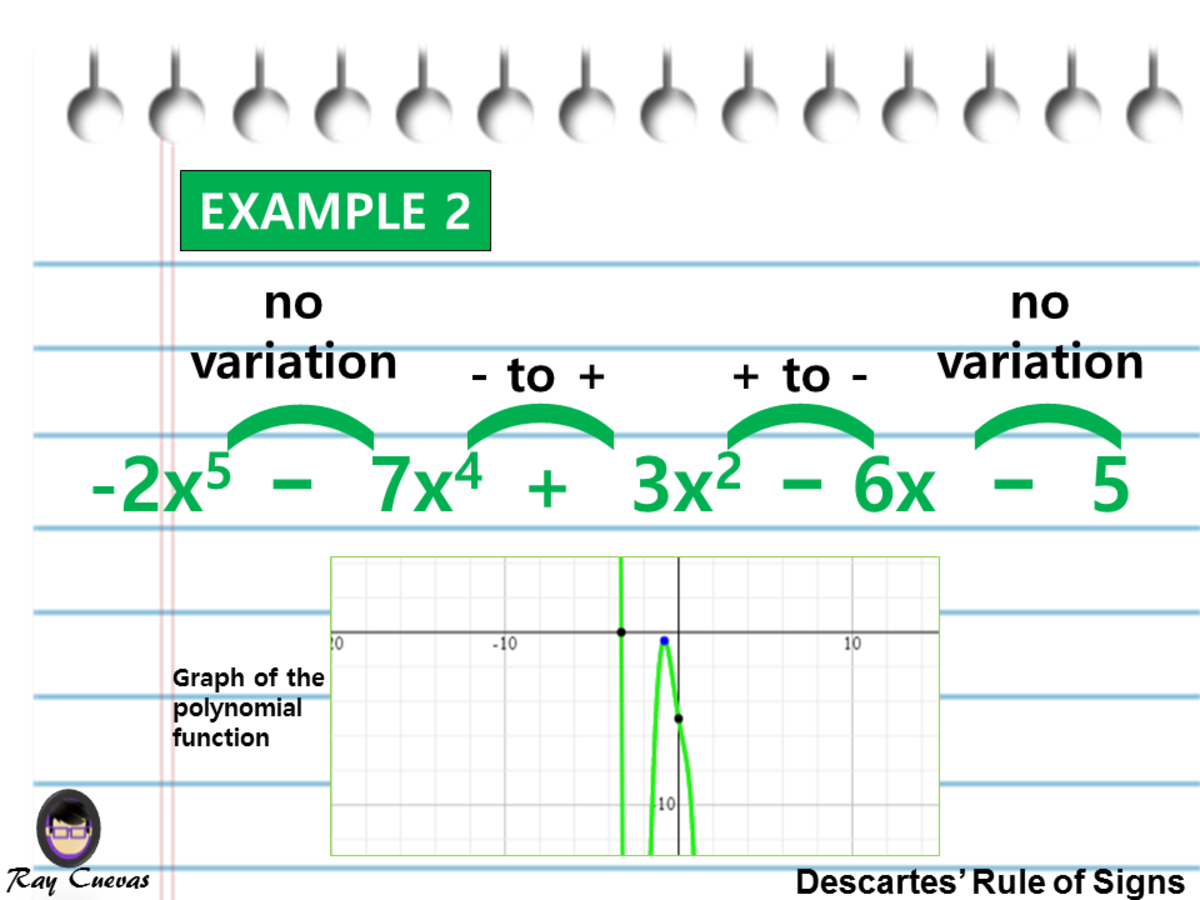Example 2: Finding the Number of Sign Variations in a Negative Polynomial Function Using Descartes' Rule of SignsJohn Ray Cuevas

## Example 3: Finding the Number of Variations in Sign of a Polynomial Function

Using the Descartes’ Rule of Signs, how many variations in sign are there in the polynomial f(x) = x4 – 3x3 + 2x2 + 3x – 5?

Solution

The signs of the terms of this polynomial arranged in descending order are shown in the image below. The figure shows the sign changes from x4 to -3x3, from -3x3 to 2x2, and from 3x to -5.

There are three variations in sign as shown by the loops above the signs.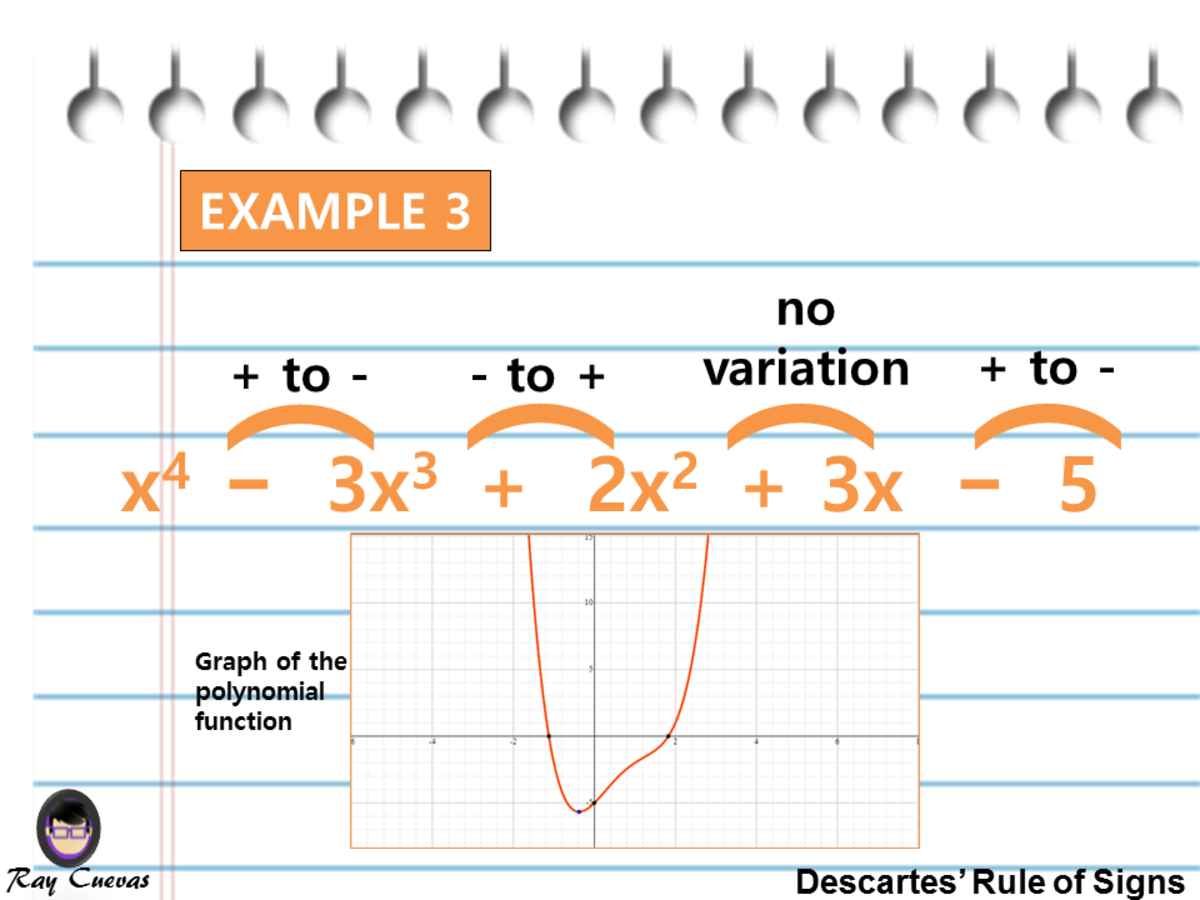Example 3: Finding the Number of Variations in Sign of a Polynomial Function Using Descartes' Rule of SignsJohn Ray Cuevas

## Example 4: Determining the Number of Possible Real Solutions to a Polynomial Function

Using the Descartes’ Rule of Signs, determine the number of real solutions to the polynomial equation 4x4 + 3x3 + 2x2 - 9x + 1.

Solution

1. The figure below shows the sign changes from 2x2 to -9x and from -9x to 1. There are two sign variations in the given polynomial equation, which mean that there are two or zero positive solutions for the equation.
2. For the negative root case f(-x), substitute –x to the equation. The image shows that there are changes in sign from 4x4 to -3x3 and -3x3 to 2x2.

There are two or zero positive real solutions. On the other hand, there are two or zero negative real solutions.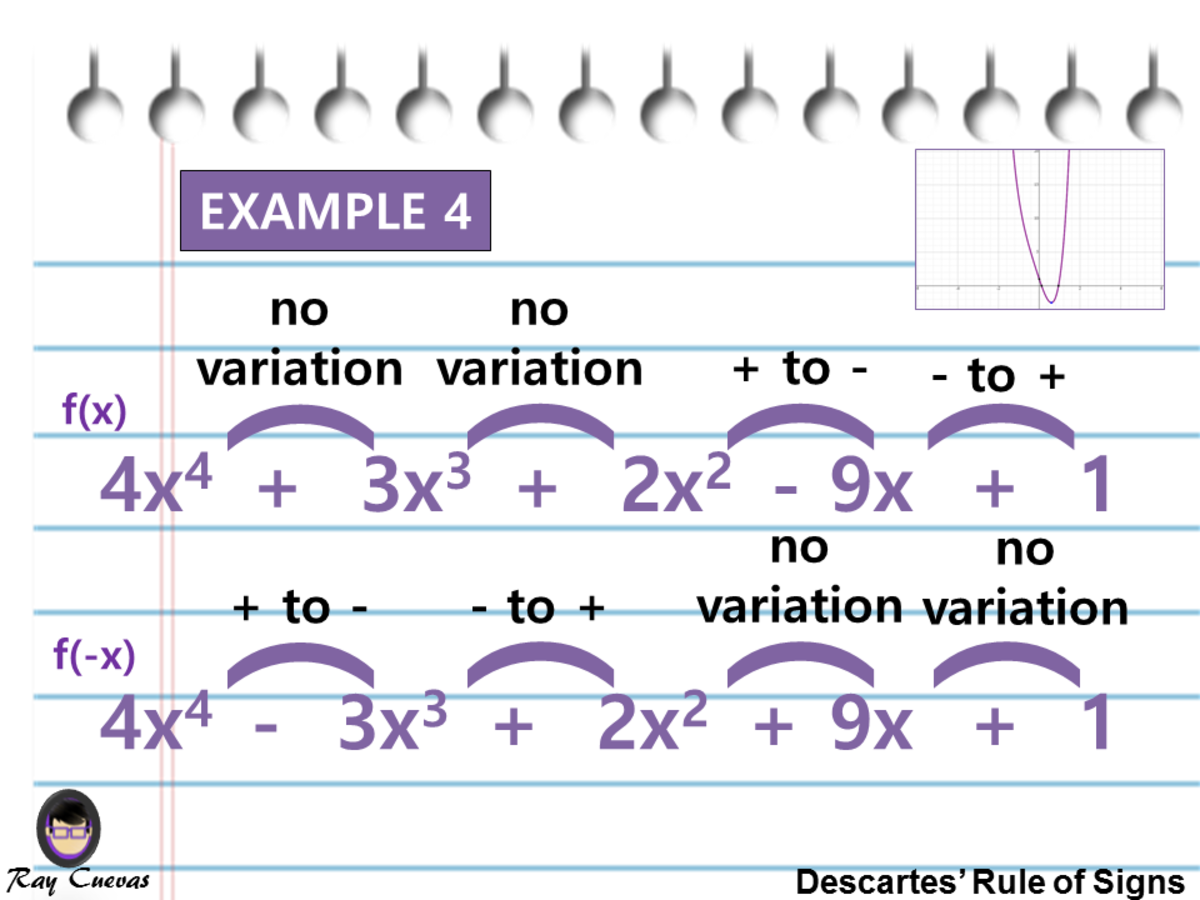Example 4: Determining the Number of Possible Real Solutions to a Polynomial Function Using Descartes' Rule of SignsJohn Ray Cuevas

## Example 5: Finding the Number of Real Roots of a Polynomial Function

Using the Descartes’ Rule of Signs, find the number of real roots of the function x5 + 6x4 - 2x2 + x − 7.

Solution

1. First assess the positive-root case by looking at the function as it is. Observe from the diagram below that the sign changes from 6x4 to -2x2, -2x2 to x, and x to -7. The signs flip three times which implicates that there are possibly three roots.
2. Next, look for the f(-x) but evaluating the negative-root case. There are sign variations from –x5 to 6x4 and 6x4 to -2x2. The signs flip twice, which means that there could be two negative roots or none at all.

Therefore, there are three positive roots or one; there are two negative roots or none at all.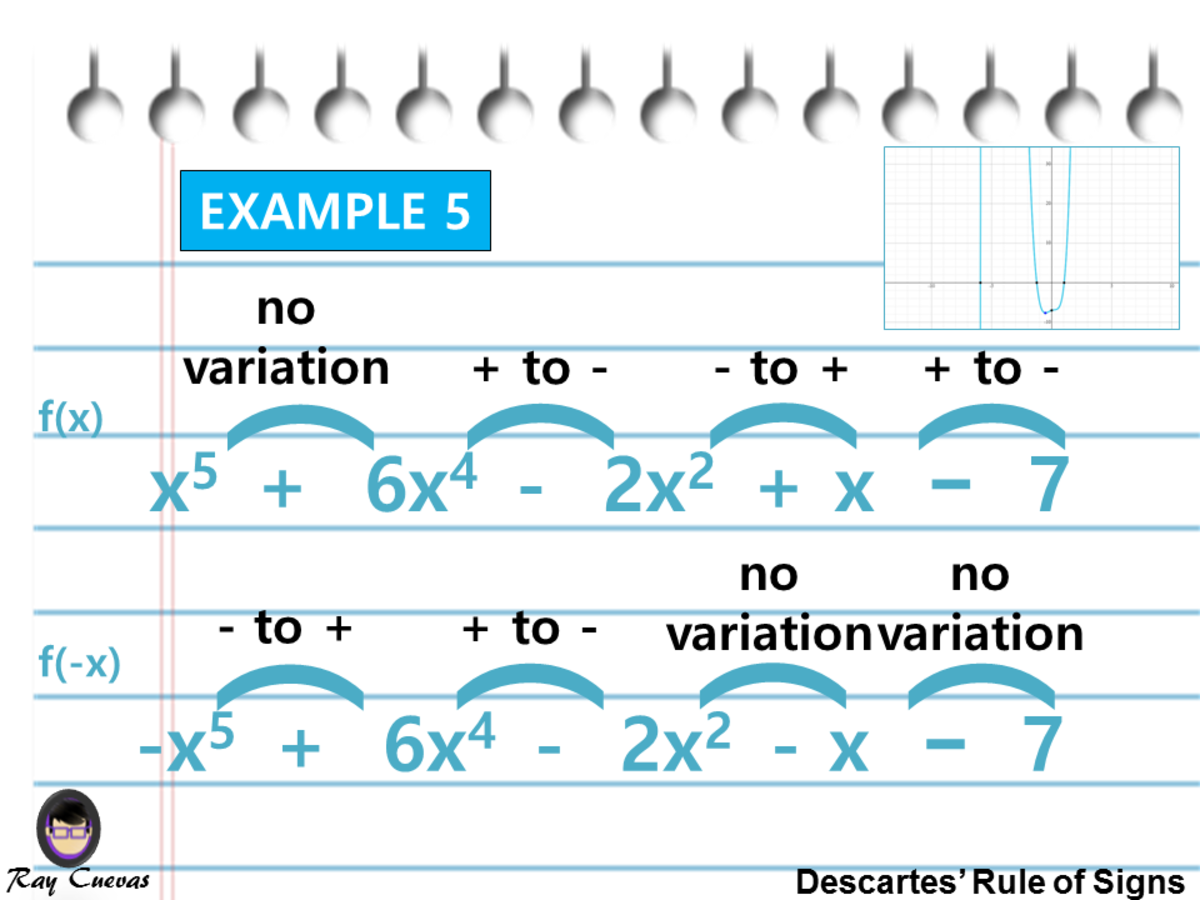Example 5: Finding the Number of Real Roots of a Polynomial Function Using Descartes' Rule of SignsJohn Ray Cuevas

## Example 6: Determining the Possible Number of Solutions to an Equation

Determine the possible number of solutions to the equation x3 + x2 - x − 9 using the Descartes’ Rule of Signs.

Solution

1. Evaluate the function first as it is by observing the sign changes. Observe from the diagram that there is a change of sign from x2 to –x only. The signs change once, which suggests that the function has exactly one positive root.
2. Assess the negative-root case by counting on the sign variations for f(-x). As you can see from the image, there are sign switches from –x3 to x2 and x to -9. The sign switches show that equation either have two negative roots or none at all.

Therefore, there is exactly one positive real root; there are two negative roots or none at all.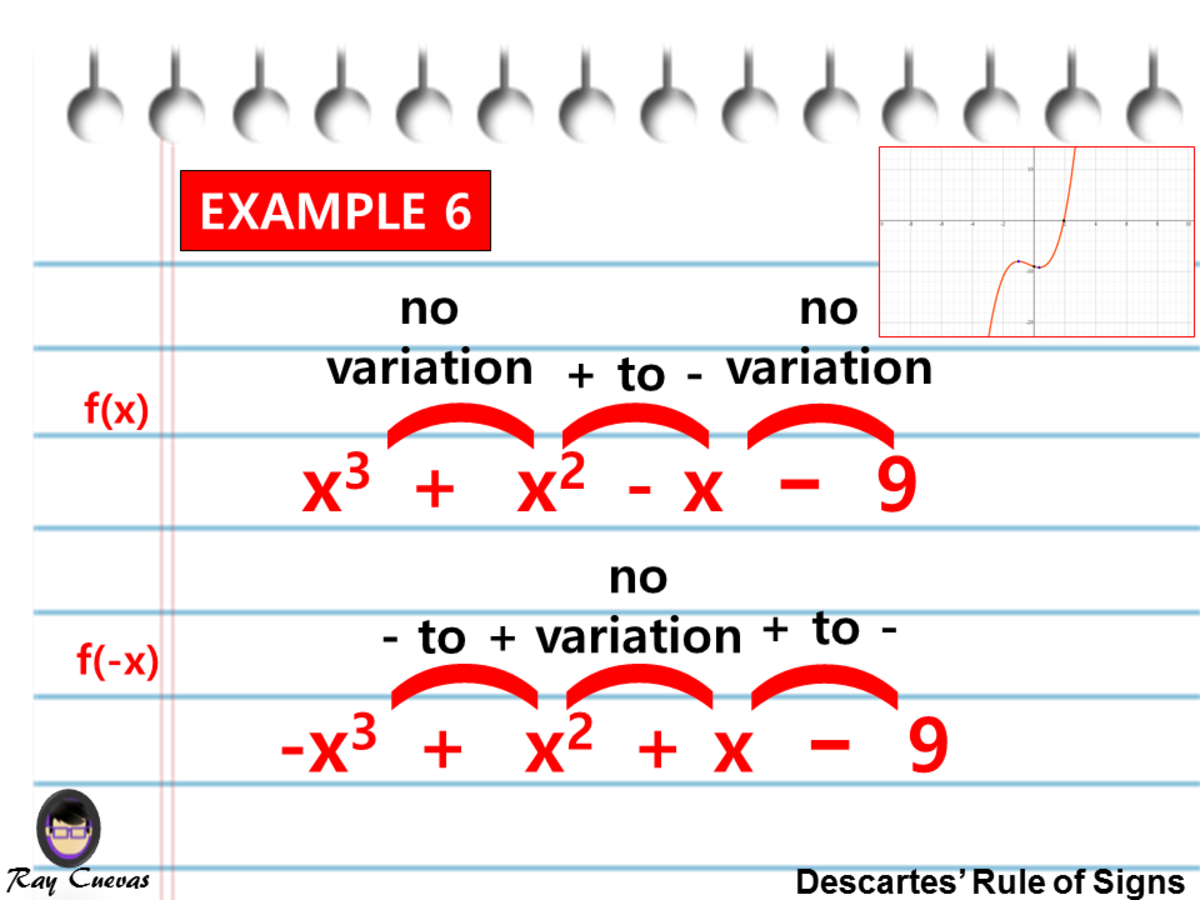Example 6: Determining the Possible Number of Solutions to an Equation Utilizing Descartes' Rule of SignsJohn Ray Cuevas

## Example 7: Determining the Number of Positive and Negative Real Solutions of a Polynomial Function

Discuss the number of possible positive and negative real solutions and imaginary solutions of the equation f(x)=0, where f(x) = 2x5 – 7x4 + 3x2 + 6x – 5.

Solution

The polynomial f(x) is the one given in the two previous examples (refer to from the earlier examples). Since there are three variations of sign in f(x), the equation has either three positive real solutions or one real positive solution.

Since f(−x) has two variations of the sign, the equation has either two negative solutions or no negative solutions or no negative solution.

Because f(x) has degree 5, there are a total of 5 solutions. The solutions that are not positive or negative real numbers are imaginary numbers. The following table summarizes the various possibilities that can occur for solutions of the equation.

Table 1: Descartes’ Rule of Signs. This table shows the number of positive real solutions, negative real solutions, and imaginary solutions for the given function.

Number of Positive Real SolutionsNumber of Negative Real SolutionsNumber of Imaginary SolutionsTotal Number of Solutions

3

2

0

5

3

0

2

5

1

2

2

5

1

0

4

5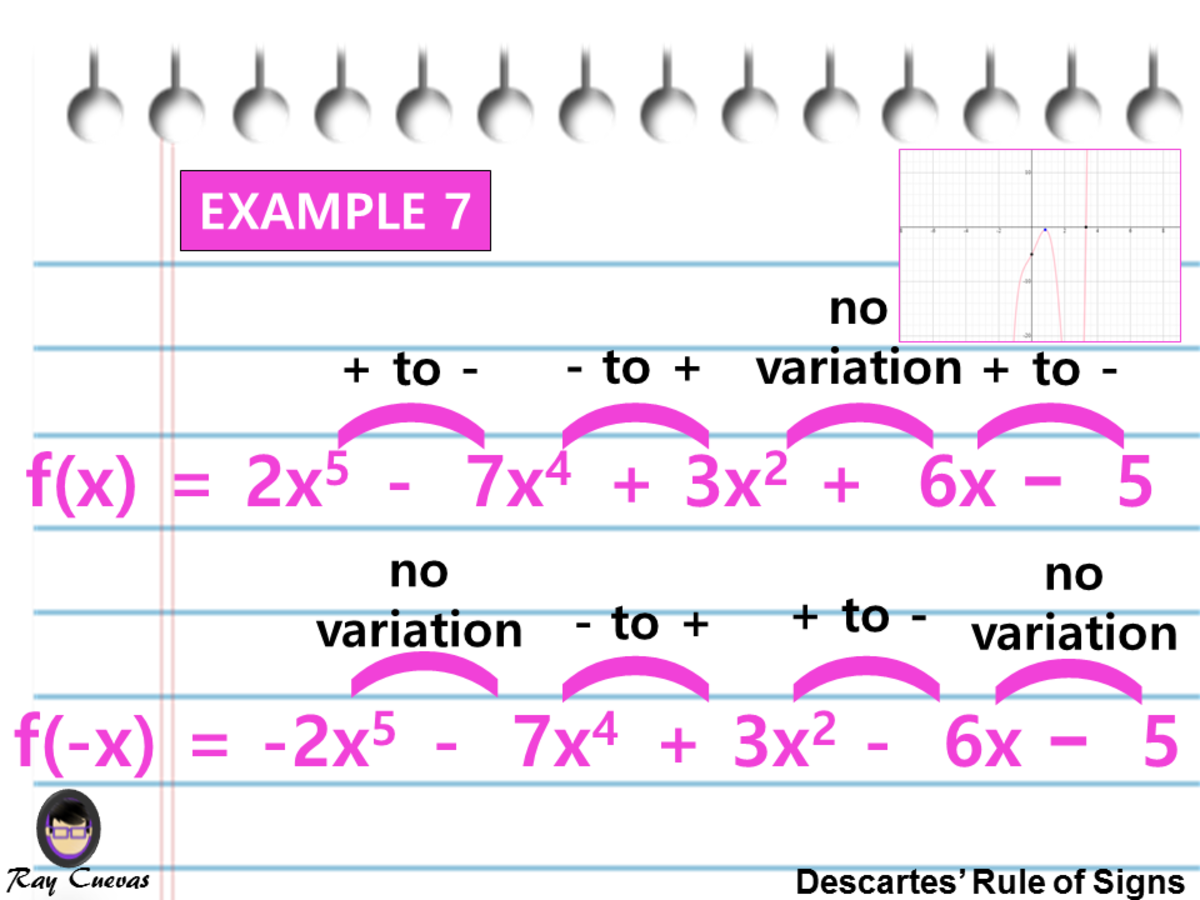Example 7: Determining the Number of Positive and Negative Real Solutions of a Polynomial FunctionJohn Ray Cuevas

## Example 8: Determining the Number of Positive and Negative Roots of a Function

Determine the nature of the roots of the polynomial equation 2x6 + 5x2 - 3x + 7 = 0 using Descartes’ Rule of Signs.

Solution

Let P(x) = 2x6 + 5x2 − 3x + 7. First, identify the number of variations in the sign of the given polynomial using the Descartes’ Rule of Signs. The signs of the terms of this polynomial arranged in descending order are shown below given that P(x) = 0 and P(−x) = 0.

There are two positive roots or 0 positive roots. Also, there are no negative roots. The possible combinations of roots are:

Table 2: Descartes’ Rule of Signs. This table shows the number of positive roots, negative roots, and non-real roots of the given function.

Number of Positive RootsNumber of Negative RootsNumber of Non-Real RootsTotal Number of Solutions

2

0

4

6

0

0

6

6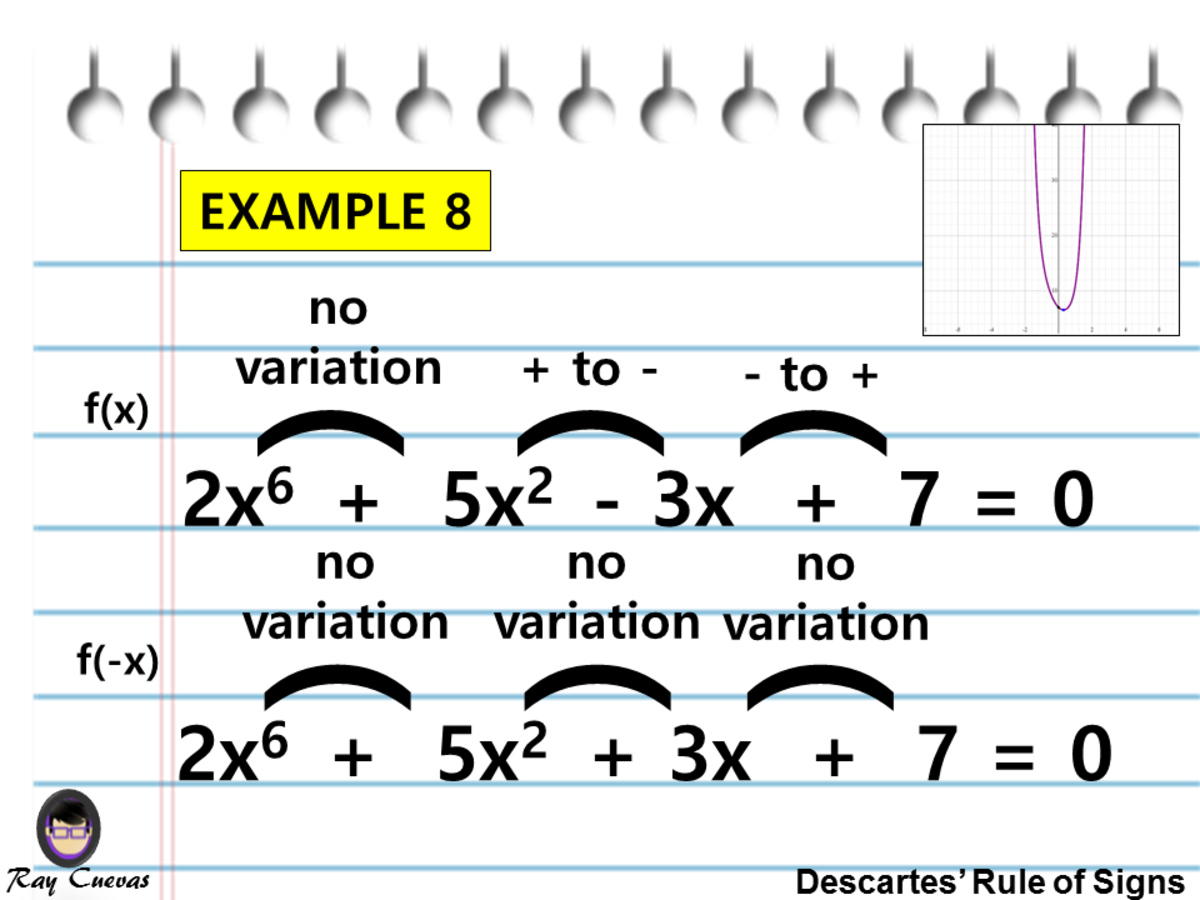Example 8: Determining the Number of Positive and Negative Roots of a FunctionJohn Ray Cuevas

## Example 9: Identifying the Possible Combination of Roots

Determine the nature of the roots of the equation 2x3 - 3x2 - 2x + 5 = 0.

Solution

Let P(x) = 2x3 − 3x2 − 2x + 5. First, identify the number of variations in the sign of the given polynomial using the Descartes’ Rule of Signs. The signs of the terms of this polynomial arranged in descending order are shown below given that P(x) = 0 and P(−x) = 0.

The possible combinations of roots are:

Table 3: Descartes’ Rule of Signs. This table shows the number of positive roots, negative roots, and non-real roots of the given function.

Number of Positive RootsNumber of Negative RootsNumber of Non-Real RootsTotal Number of Solutions

2

1

0

3

0

1

2

3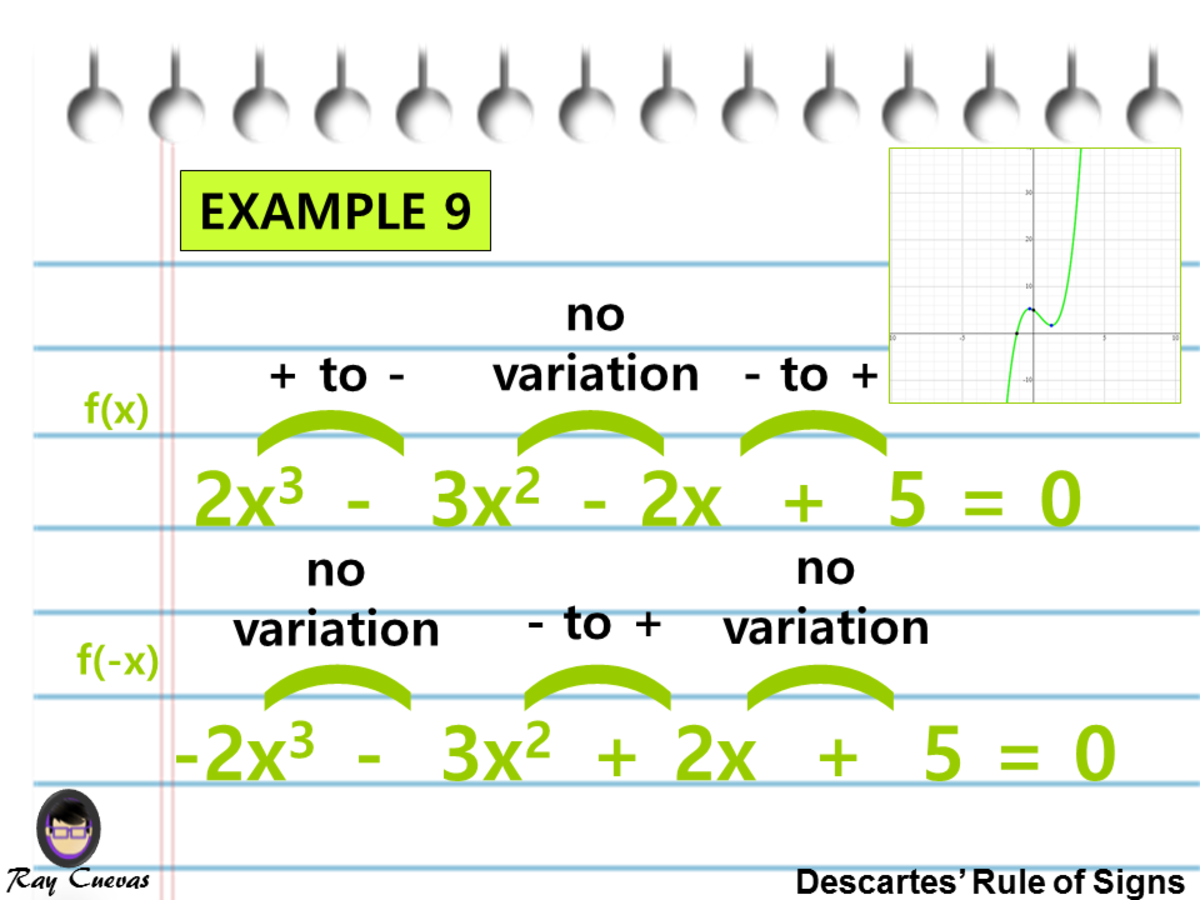Example 9: Identifying the Possible Combination of RootsJohn Ray Cuevas

## Explore Other Math Articles

This content is accurate and true to the best of the author’s knowledge and is not meant to substitute for formal and individualized advice from a qualified professional.